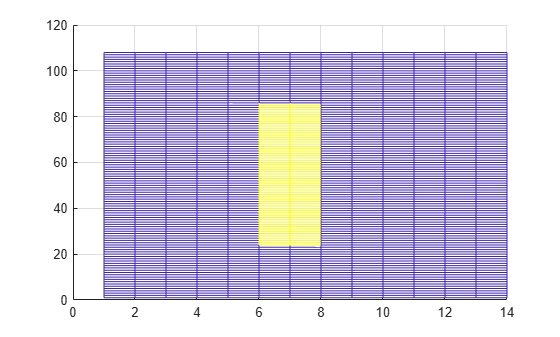Main Content

# Create Synchronization Signals

This example shows how to construct synchronization signals using LTE Toolbox™. In this example, you create the primary and secondary synchronization signals and map them to a resource grid.

Set up the cell-wide settings. Create a structure and specify the cell-wide settings as its fields.

```enb.NDLRB = 9; enb.CyclicPrefix = 'Normal'; enb.CellRefP = 1; enb.NCellID = 1; enb.NSubframe = 0; enb.DuplexMode = 'FDD';```

Many of the functions used in this example require a subset of the preceding settings specified.

Generate the PSS symbols bu calling the `ltePSS` function with the cell-wide settings specified by `enb`.

`pss = ltePSS(enb);`

When a PSS signal is not located in `enb.NSubframe`, the function does not generate PSS symbols and returns an empty vector.

Next, generate the PSS indices. These indices map the PSS complex symbols to the subframe resource grid. Use the `ltePSSIndices` function for the specified cell-wide settings and antenna number. In this case, since only one antenna port is used, specify `antenna` as `0`.

```antenna = 0; pssIndices = ltePSSIndices(enb, antenna);```

In this example, you generate subframe 0. Since subframe 0 contains a PSS signal, the function generates PSS indices. If `enb.NSubframe` is a subframe that does not contain a PSS signal, the function would return an empty vector.

Generate the SSS symbols by calling the `lteSSS` function with the cell-wide settings specified by `enb`.

`sss = lteSSS(enb);`

When an SSS signal is not located in `enb.NSubframe`, the function does not generate SSS symbols. It returns an empty vector.

Next, generate the SSS indices. These indices map the SSS complex symbols to the subframe resource grid. Call the `lteSSSIndices` function, providing the cell-wide settings `enb` and the antenna port number `antenna`.

```antenna = 0; sssIndices = lteSSSIndices(enb, antenna);```

In this example, you generate subframe 0. Since subframe 0 contains an SSS signal, the function generates SSS indices. If `enb.NSubframe` is a subframe that does not contain an SSS signal, the function returns empty indices.

Generate the subframe resource grid by calling the `lteDLResourceGrid` function. You create an empty resource grid for one subframe.

`subframe = lteDLResourceGrid(enb);`

Finally, map the PSS and SSS symbols directly to the resource grid using the generated indices. Show the synchronization symbols mapped in RE grid.

```subframe(pssIndices) = pss; subframe(sssIndices) = sss; mesh(abs(subframe)) view(2)```